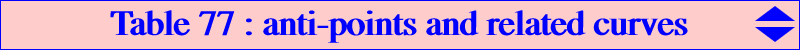Sources : • Arthur Cayley : Antipoints, Definition and Fundamental Properties. Collected Mathematical Papers, Volume VI, Article No. 63, p. 499. • Charles Edward Brooks : Orthic Curves; Or, Algebraic Curves Which Satisfy Laplace's Equation in Two Dimensions. Proceedings of the American Philosophical Society, Vol. 43, No. 177 (Apr. - Sep., 1904), pp. 294-331. *** The cevian lines of the circular points at infinity J1, J2 meet at A, B, C and 3 pairs of imaginary points called the anti-points (of triangle ABC). One pair is A1 = BJ2 /\ CJ1 = -a^2 : SC + i S : SB + i S and A2 = BJ1 /\ CJ2 = -a^2 : SC - i S : SB - i S, where S = 2 area(ABC). The other pairs {B1, B2} and {C1, C2} are defined cyclically. Properties of these points A1 and A2 are isogonal conjugates on the perpendicular bisector of BC, hence they lie on K003. A1 and A2 must lie on the isogonal tranform of the perpendicular bisector of BC. This is the rectangular circum-hyperbola passing through the antipode of A on (O). Its center is the midpoint of BC and the tangent at A is the A-symmedian. A1 and A2 are symmetric about the line BC and their midpoint is that of BC. If Ωa, Ωb, Ωc denote the centers of the Apollonius circles (these points are the traces of the Lemoine axis on the sidelines of ABC), then B1C1 and B2C2 meet at Ωa. Note that A1, A2 lie on the A-Apollonius circle with center Ωa. *** Circum-cubics passing through the anti-points These form a pencil of stelloids which contains K003, K024, K213. See Table 22. *** Circular cubics passing through the anti-points These also form a pencil of cubics passing through O and inversible in the circumcircle. The singular focus F lies on (O) and it is the antipode of the isogonal conjugate of the real point at infinity. The real asymptote (A) is tangent to the deltoid which is obtained from aH3 under the translation that sends O to H or, equivalently, from H3 under the homothety h(X381, -2). The tangent at O is parallel to (A) and the orthic line (L) is the parallel at X(5) to (A). The cubic is a K+ if and only if it passes through one infinite point of pK(X6, X550). In this case, the asymptote passes through X(5) and F. The cubic is a proper axial cubic if and only if it passes through one infinite point of K024. Examples : the Apollonian strophoids K053A, K053B, K053C (the isogonal transforms of the Apollonius circles) and the focal cubics K110A, K110B, K110C (the isogonal transforms of the focal pKs with pivot H). *** Other cubics passing through the anti-points See K727, K1249, K1250 which meet the circumcircle at the same points as K002, K102, K006 and the line at infinity at the same points as another isogonal pK. More generally, for P on K002, there is a cubic K(P) passing through the anti-points and the points O1, O2, O3 (other than A, B, C) of pK(X6, P) on (O). In this case, K(P) meets (O) again at the antipodes Q1, Q2, Q3 of the common points R1, R2, R3 (apart A, B, C) of (O) and pK(X6, Q) where Q is the Cundy-Parry Psi-transform of P, hence Q lies on K004. These points Q1, Q2, Q3 also lie on an isogonal nK0 whose root lies on K1252. *** Other curves of higher degree passing through the anti-points Q019, Q021, Q024, Q025, Q030, Q037, Q039, Q047, Q050, Q064, Q085, Q086, Q094, Q125, Q126, Q127, Q128, Q129, Q130, Q131, Q132, Q133, Q134, Q136, Q139, Q152, Q169, Q170, Q171, Q185, Q186. See also the sextics mentioned in Q170. Note that almost all the curves above are either bicircular / circular or stelloids.